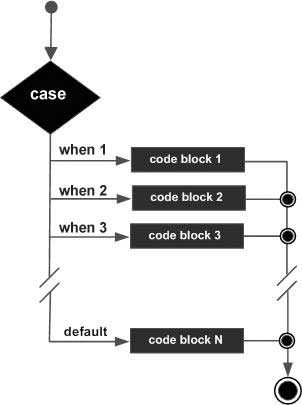# PL/SQL - Searched CASE Statement

Advertisements

The searched CASE statement has no selector and the WHEN clauses of the statement contain search conditions that give Boolean values.

## Syntax

The syntax for the searched case statement in PL/SQL is −

```CASE
WHEN selector = 'value1' THEN S1;
WHEN selector = 'value2' THEN S2;
WHEN selector = 'value3' THEN S3;
...
ELSE Sn;  -- default case
END CASE;
```

## Flow Diagram## Example

```DECLARE
grade char(1) := 'B';
BEGIN
case
when grade = 'A' then dbms_output.put_line('Excellent');
when grade = 'B' then dbms_output.put_line('Very good');
when grade = 'C' then dbms_output.put_line('Well done');
when grade = 'D' then dbms_output.put_line('You passed');
when grade = 'F' then dbms_output.put_line('Better try again');
else dbms_output.put_line('No such grade');
end case;
END;
/
```

When the above code is executed at the SQL prompt, it produces the following result −

```Very good

PL/SQL procedure successfully completed.
```
plsql_conditional_control.htm
Advertisements